• Batch Normalization 和 Batch Renormalization 前向和反向公式详细推导 文章目录Batch Normalization 和 Batch Renormalization 前向和反向公式详细推导一、BN前向传播二、BN的反向传播三、Batch Renormalization1...
Batch Normalization 和 Batch Renormalization 前向和反向公式详细推导

CSDN的Latex引擎好像有点问题，有些语句刷不出来，，，如有需要可以看我的博客园同文https://www.cnblogs.com/lyc-seu/p/12676505.html

文章目录Batch Normalization 和 Batch Renormalization 前向和反向公式详细推导一、BN前向传播二、BN的反向传播三、Batch Renormalization1、前向2、反向三、卷积网络中的BN
一、BN前向传播
根据论文‘’Batch Normalization: Accelerating Deep Network Training by Reducing Internal Covariate Shift" 的推导过程，主要有下面四个公式：
$\mu_B=\frac{1}{m}\sum_i^mx_i\tag{1}$
$\delta_B^2=\frac{1}{m}\sum_i^m(x_i-\mu_B)^2\tag{2}$
$\widehat{x_i}=\frac{x_i-\mu_B}{\sqrt{\delta_B^2+\epsilon}}\tag{3}$
$y_i=\gamma\widehat{x_i}+\beta\tag{4}$
以MLP为例，假设输入的mini-batch样本数为$m$，则此处的$x_i,i=1,2,...m$是第$i$个样本对应的某一层激活值中的一个激活值。也就是说，假设输入$m$个样本作为一次训练，其中第$i$个样本输入网络后，在$l$层得到了$N$个激活单元，则$x_i$代表其中任意一个激活单元。事实上应该写为$x_i^l(n)$更为直观。
所以BN实际上就是对第$l$层的第$n$个激活单元$x_i^l(n)$求其在一个batch中的平均值和方差，并对其进行标准归一化，得到$\widehat{x_i^l(n)}$，可知归一化后的m个激活单元均值为0方差为1，一定程度上消除了Internal Covariate Shift，减少了网络的各层激活值在训练样本上的边缘分布的变化。
二、BN的反向传播

设前一层的梯度为$\frac{\partial{L}}{\partial{y_i}}$.
需要计算$\frac{\partial{L}}{\partial{x_i}},\frac{\partial{L}}{\partial{\gamma}}以及\frac{\partial{L}}{\partial{\beta}}$

由链式法则以及公式\eqref{4}：
$\frac{\partial{L}}{\partial{\gamma}}=\frac{\partial{L}}{\partial{y_i}}\frac{\partial{y_i}}{\partial{\gamma}}=\frac{\partial{L}}{\partial{y_i}}\widehat{x_i} \tag{5}$
由于对于所有$i=1,2...m. \frac{\partial{L}}{\partial{y_i}}\widehat{x_i}对\frac{\partial{L}}{\partial{\gamma}}$均有贡献，因此一个batch的训练中将$\frac{\partial{L}}{\partial{\gamma}}$定义为：
$\frac{\partial{L}}{\partial{\gamma}}=\sum_{i=1}^m \frac{\partial{L}}{\partial{y_i}}\widehat{x_i}\tag{6}$
同样有：
$\frac{\partial{L}}{\partial{\beta}}=\sum_{i=1}^m \frac{\partial{L}}{\partial{y_i}}\tag{7}$
另外，求$\frac{\partial{L}}{\partial{x_i}}$过程则较为复杂。根据链式法则，以及公式KaTeX parse error: Undefined control sequence: \eqref at position 1: \̲e̲q̲r̲e̲f̲{3},将$\widehat{x_i}$视为$g(x_i,\delta_B^2,\mu_B)$有：
$\frac{\partial{L}}{\partial{x_i}}=\frac{\partial{L}}{\partial{y_i}}\frac{\partial{y_i}}{\partial{\widehat{x_i}}}(\frac{\partial{\widehat{x_i}}}{\partial{x_i}}+\frac{\partial{\widehat{x_i}}}{\partial{\delta_B^2}}\frac{\partial{\delta_B^2}}{\partial{x_i}}+\frac{\partial{\widehat{x_i}}}{\partial{\mu_B}}\frac{\partial{\mu_B}}{\partial{x_i}}) =\frac{\partial{L}}{\partial{y_i}}\frac{\partial{y_i}}{\partial{\widehat{x_i}}}(g_1'+g_2'\frac{\partial{\delta_B^2}}{\partial{x_i}}+g_3'\frac{\partial{\mu_B}}{\partial{x_i}}) \tag{8}$
而因为公式KaTeX parse error: Undefined control sequence: \eqref at position 1: \̲e̲q̲r̲e̲f̲{2}可知上式括号中的第二项求偏导可以进一步拆分。（将$\delta_B^2$视为$f(x_i,\mu_B)$）
$\frac{\partial{\delta_B^2}}{\partial{x_i}}= \frac{\partial{\delta_B^2}}{\partial{x_i}}+ \frac{\partial{\delta_B^2}}{\partial{\mu_B}} \frac{\partial{\mu_B}}{\partial{x_i}}= f_1'+f_2'\frac{\partial{\mu_B}}{\partial{x_i}} \tag{9}$
注意公式KaTeX parse error: Undefined control sequence: \eqref at position 1: \̲e̲q̲r̲e̲f̲{9}中的两个$\frac{\partial{\delta_B^2}}{\partial{x_i}}$代表不同的含义。由公式KaTeX parse error: Undefined control sequence: \eqref at position 1: \̲e̲q̲r̲e̲f̲{8},\eqref{9}可知，只要求出$f_1',f_2',g_1',g_2',g_3',\frac{\partial{\mu_B}}{\partial{x_i}},\frac{\partial{y_i}}{\partial{\widehat{x_i}}}$.即可求出$\frac{\partial{L}}{\partial{x_i}}$.
原论文中将公式KaTeX parse error: Undefined control sequence: \eqref at position 1: \̲e̲q̲r̲e̲f̲{8}拆分成如下几项:
$\frac{\partial{L}}{\partial{x_i}}= \frac{\partial{L}}{\partial{\widehat{x_i}}} \frac{\partial{\widehat{x_i}}}{\partial{x_i}}+ \frac{\partial{L}}{\partial{\delta_B^2}} \frac{\partial{\delta_B^2}}{\partial{x_i}}+ \frac{\partial{L}}{\partial{\mu_B}} \frac{\partial{\mu_B}}{\partial{x_i}} \tag{10}$
其中：
$\frac{\partial{L}}{\partial{\widehat{x_i}}}= \frac{\partial{L}}{\partial{y_i}} \frac{\partial{y_i}}{\partial{\widehat{x_i}}}= \frac{\partial{L}}{\partial{y_i}} \gamma\tag{10.1}$
$\frac{\partial{\widehat{x_i}}}{\partial{x_i}}=g'_1=\frac{1}{\sqrt{\delta_B^2+\epsilon}} \tag{10.2}$
$\frac{\partial{L}}{\partial{\delta_B^2}}= \frac{\partial{L}}{\partial{\widehat{x_i}}}g'_2= \frac{\partial{L}}{\partial{\widehat{x_i}}} \frac{\mu_B-x_i}{2}(\delta_B^2+\epsilon)^{-\frac{3}{2}} \longrightarrow$
$\sum_{i=1}^m\frac{\partial{L}}{\partial{\widehat{x_i}}} \frac{\mu_B-x_i}{2}(\delta_B^2+\epsilon)^{-\frac{3}{2}} \tag{10.3}$
$\frac{\partial{\delta_B^2}}{\partial{x_i}}=f'_1=\frac{2(x_i-\mu_B)}{m} \tag{10.4}$
$\frac{\partial{L}}{\partial{\mu_B}}= \frac{\partial{L}}{\partial{\widehat{x_i}}}g'_3+ \frac{\partial{L}}{\partial{\widehat{x_i}}}g'_2f'_2 \longrightarrow$
$\sum_{i=1}^m( \frac{\partial{L}}{\partial{\widehat{x_i}}}\frac{-1}{\sqrt{\delta_B^2+\epsilon}} +\frac{\partial{L}}{\partial{\delta_B^2}}\frac{2(\mu_B-x_i)}{m}) \tag{10.5}$
$\frac{\partial{\mu_B}}{\partial{x_i}}=\frac{1}{m} \tag{10.6}$
最终BN的反向过程由公式KaTeX parse error: Undefined control sequence: \eqref at position 1: \̲e̲q̲r̲e̲f̲{6},\eqref{7},\…给出。
三、Batch Renormalization

参照论文—— Batch Renormalization: Towards Reducing Minibatch Dependence
in Batch-Normalized Models

Batch Renormalization是对传统BN的优化，该方法保证了train和inference阶段的等效性，解决了非独立同分布和小minibatch的问题。
1、前向
跟原来的公式类似，添加了两个非训练参数$r,d$:
$\mu_B=\frac{1}{m}\sum_i^mx_i\tag{1.1}$
$\sigma_B=\sqrt{\epsilon+\frac{1}{m}\sum_i^m(x_i-\mu_B)^2}\tag{2.1}$
$\widehat{x_i}=\frac{x_i-\mu_B}{\sigma_B}r+d\tag{3.1}$
$y_i=\gamma\widehat{x_i}+\beta\tag{4.1}$
$r=Stop\_Gradient(Clip_{[1/r_{max} ,r_{max}]}(\frac{\sigma_B}{\sigma}))\tag{5.1}$
$d=Stop\_Gradient(Clip_{[-d_{max} ,d_{max}]}(\frac{\mu_B-\mu}{\sigma}))\tag{6.1}$

Update moving averages:

$\mu:=\mu+\alpha(\mu_B-\mu)\tag{7.1}$
$\sigma:=\sigma+\alpha(\sigma_B-\sigma)\tag{8.1}$

Inference:

$y=\gamma\frac{x-\mu}{\sigma}+\beta\tag{9.1}$
相比于之前的BN只在训练时计算滑动均值与方差，推断时才使用他们；BRN在训练和推断时都用到了滑动均值与方差。
2、反向
反向的推导与BN类似，
$\frac{\partial{L}}{\partial{\widehat{x_i}}}= \frac{\partial{L}}{\partial{y_i}} \frac{\partial{y_i}}{\partial{\widehat{x_i}}}= \frac{\partial{L}}{\partial{y_i}} \gamma\tag{10.11}$
$\frac{\partial{L}}{\partial{\sigma_B}} \longrightarrow\sum_{i=1}^m \frac{\partial{L}}{\partial{\widehat{x_i}}} \frac{-r(x_i-\mu_B)}{\sigma_B^2} \tag{10.22}$
$\frac{\partial{L}}{\partial{\mu_B}}\longrightarrow\sum_{i=1}^{m}\frac{\partial{L}}{\partial{\widehat{x_i}}}\frac{-r}{\sigma_B} \tag{10.33}$
$\frac{\partial{L}}{\partial{x_i}}= \frac{\partial{L}}{\partial{\widehat{x_i}}} \frac{r}{\sigma_B}+ \frac{\partial{L}}{\partial{\sigma_B}} \frac{x_i-\mu_B}{m\sigma_B}+ \frac{\partial{L}}{\partial{\mu_B}} \frac{1}{m} \tag{10.44}$
$\frac{\partial{L}}{\partial{\gamma}}=\sum_{i=1}^m \frac{\partial{L}}{\partial{y_i}}\widehat{x_i}\tag{10.55}$
$\frac{\partial{L}}{\partial{\beta}}=\sum_{i=1}^m \frac{\partial{L}}{\partial{y_i}}\tag{10.66}$
三、卷积网络中的BN
​        上面的推导过程都是基于MLP的。对于卷积网络而言，BN过程中的m个激活单元被推广为m幅特征图像。 假设某一层卷积后的feature map是$[N,H,W,C]$的张量，其中N表示batch数目，H,W分别表示长和宽，C表示特征通道数。则对卷积网络的BN操作时，令$m = N\times H\times W$,也就是说将第$i$个batch内某一通道$c$上的任意一个特征图像素点视为$x_i$,套用上面的BN公式即可。所以对于卷积网络来说，中间激活层每个通道都对应一组BN参数$\gamma,\beta$.


展开全文深度学习
• 上式带入公式(1)得到 ∂ O ∂ x i j = γ j m σ j 2 + ϵ ( σ j 2 + ϵ ) ( ( σ j 2 + ϵ ) ( m ∂ O ∂ y j j − ∑ k ∂ O ∂ y k j ) − ( x i j − μ j ) ( x k j − μ j ) ∑ k ∂ O ∂ y k j ) ( d o n...
输入
$X_i=(x_{i0},x_{i1},...,x_{i(n-1)})$ $i \in [0,m-1]$ batch-size等于m,特征维度n
输出
$Y_i=(y_{i0},y_{i1},...,y_{i(n-1)})$ $i \in [0,m-1]$ 维度和输入$X$一致
前向计算

均值
$\mu = {\mu_0,\mu_1,...,\mu_n}$ 其中
$\mu_p = \frac{1}{m}\sum_ix_{ip}$

方差
$\sigma = {\sigma_0,\sigma_1,...,\sigma_n}$ 其中
$\sigma_p = \frac{1}{m}\sum_i(x_{ip}-\mu_p)^2$

中间结果
$\overline x_{ip}=\frac{x_{ip}-\mu_p}{\sqrt{\sigma_p^2+\epsilon}}$

结果
$y_{ip}=\gamma_p \overline x_{ip}+\beta_p$ 其中
参数$\gamma = {\gamma_0, \gamma_1,...,\gamma_{n-1}}$  和
$\beta = {\beta_0,\beta_1,...,\beta_{n-1}}$
是learnable parameters

反向计算
$\frac{\partial O}{\partial x_{ij}}=\sum_{kl}{ \frac{\partial O}{\partial y_{kl}} } \frac{\partial y_{kl}}{\partial x_{ij}} = \sum_{kl}{ \frac{\partial O}{\partial y_{kl}} } \frac{\partial y_{kl}}{\partial \overline x_{ij}} \frac{\partial \overline x_{ij}}{\partial x_{ij}} = \sum_{kl}{ \frac{\partial O}{\partial y_{kl}} } \gamma_l \frac{\partial \overline x_{ij}}{\partial x_{ij} } \quad (1)$
$\frac{\partial \overline x_{ij}}{\partial x_{ij}} = \frac { \frac{\partial{ (x_{kl}-\mu_l)}}{\partial x_{ij}} \sqrt{\sigma_l^2+\epsilon} - \frac{ \partial {\sqrt{\sigma_l^2+\epsilon}} }{\partial x_{ij}}(x_{kl}-\mu_l) } { \sigma_l^2+\epsilon } \quad (2)$
$\frac{ \partial (x_{kl}-\mu_l)}{\partial x_{ij}} = \delta_{ki}\delta_{lj} - \delta_{lj} \frac{1}{m} \quad (3)$
其中
$\delta_{pq}= \begin{cases} 1 \quad p=q \\ 0 \quad else \end{cases}$
这个符号可以替代推导过程中的if-else，遇到求和号可以消除
$\frac{\partial \sqrt{\sigma_l^2 + \epsilon}} {\partial x_{ij}} = \frac{1}{m} \frac{1}{\sqrt{\sigma_l^2+\epsilon}} \delta_{lj} (x_{il} - \mu_l) \quad (4)$
(3)(4)带入(2)得到
$\frac{\partial \overline x_{ij}}{\partial x_{ij}} = \delta_{lj} \frac { (\delta_{ki} - \frac{1}{m}) \sqrt{\sigma_l^2 + \epsilon} - \frac{1}{m\sqrt{\sigma_l^2 + \epsilon}}(x_{kl}-\mu_l)(x_{il}-\mu_l) } {\sigma_l^2 + \epsilon}$
上式带入公式(1)得到
$\frac{\partial O}{\partial x_{ij}} = \frac{\gamma_j}{m\sqrt{\sigma_j^2 + \epsilon}(\sigma_j^2 + \epsilon)} ( (\sigma_j^2 + \epsilon)( m\frac{\partial O}{\partial y_{jj}}-\sum_k\frac{\partial O}{\partial y_{kj}}) - (x_{ij}-\mu_j)(x_{kj}-\mu_j)\sum_k\frac{\partial O}{\partial y_{kj}} ) \quad (done)$


展开全文• ![图片说明](https://img-ask.csdn.net/upload/202007/01/1593590991_395496.jpg)![图片说明](https://img-ask.csdn.net/upload/202007/01/1593591007_404202.jpg)!... 求详细过程，后面的推导做不出来人工智能 神经网络 深度学习 python
• 4. 神经网络反向公式推导 (1). 权值与阈值更新公式：  (f7) (2). 输出层权值和阈值更新量反向计算：   权值w311的更新值为：    (f8) 由上式可见，权值w311是神经...
1.     神经网络架构及符号说明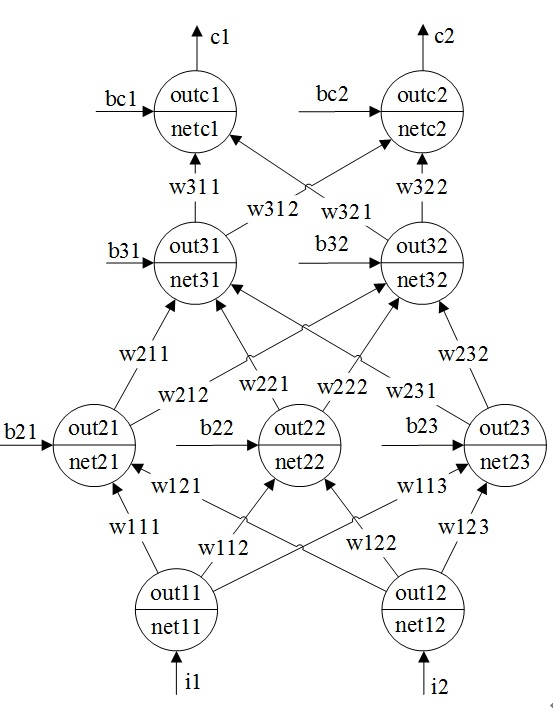图1 神经网络架构图(f1)
图1为四层神经网络的架构图，包含一个输入层(1层)，两个隐层(2,3层)，一个输出层(c层)，分别包含2个，3个，2个，2个神经元。训练的输入输出数据共包含m组样本数据。
i1和i2代表两个输入信号值。
c1和c2代表两个输出信号值。
netln代表某个神经元的输入信号之和，其下角标包含l和n两个编号，l代表这个net对应的神经元位于神经网络的第几层；n代表这个神经元是这层的第几个神经元。
outln代表某个神经元的输出信号，其下角标包含l和n两个编号，l代表这个输出信号对应的神经元位于神经网络的第几层；n代表这个神经元是这层的第几个神经元。
bln代表某个神经元的阈值，其下角标包含l和n两个编号，l代表这个阈值对应的神经元位于神经网络的第几层；n代表这个神经元是这层的第几个神经元。
wlnd代表神经网络内不同神经元之间连接的权值；其下角标包含l，n和d三个编号，l代表这个权值的输入信号的神经元位于神经网络的第几层；n代表这个神经元是这层神经元的第几个神经元；d代表这个权值的目标神经元在所在神经元层的编号。
对于每个神经元，其输入值之和经过激活函数处理之和，成为其输出值，即：outln=f(netln)
其中，f(.)代表神经元的激活函数。在本文中去sigmoid函数，但是也可以去relu函数等其它激活函数。f’(.)代表激活函数的导数。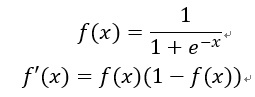(f2)
2.     神经网络前向计算公式推导
第一层：
out11=net11=i1
out12=net12=i2
即第一层的神经元为输入神经元，没有激活函数。
第二层：
net21=w111*out11+w121*out12+b21*1
net22=w112*out11+w122*out12+b22*1
net23=w113*out11+w123*out12+b23*1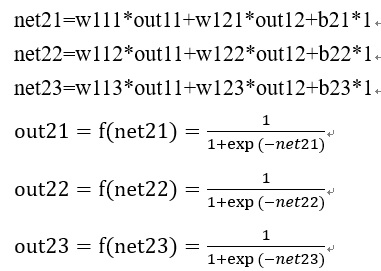(f3)
第三层：
Net31=w211*out21+w221*out22+w231*out23+b31*1
Net32=w212*out21+w222*out22+w232*out23+b32*1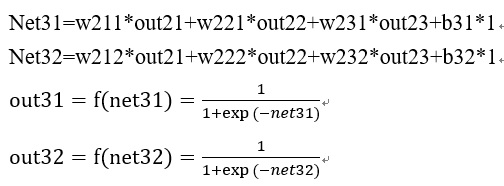(f4)
输出层：
Netc1=w311*out31+w321*out32+bc1*1
Netc2=w312*out31+w322*out32+bc2*1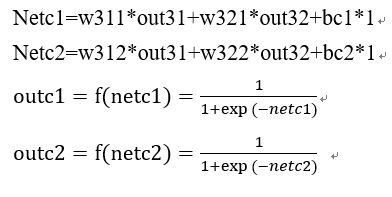(f5)
3.     代价函数
如果神经网络训练的目标是连续值，那么采用欧式距离函数来对其进行训练。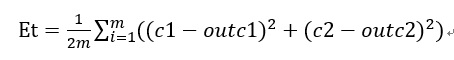(f6)
其中m代表m组训练数据。
如果神经网络训练的目标是进行分类，那么采用softmaxloss代价函数来对神经网络进行训练。本文以欧式距离函数为例来进行说明，softmaxloss代价函数可参考其它文档。
4.     神经网络反向公式推导
(1).  权值与阈值更新公式：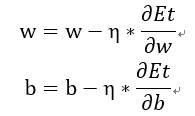(f7)
(2).  输出层权值和阈值更新量反向计算：

权值w311的更新值为：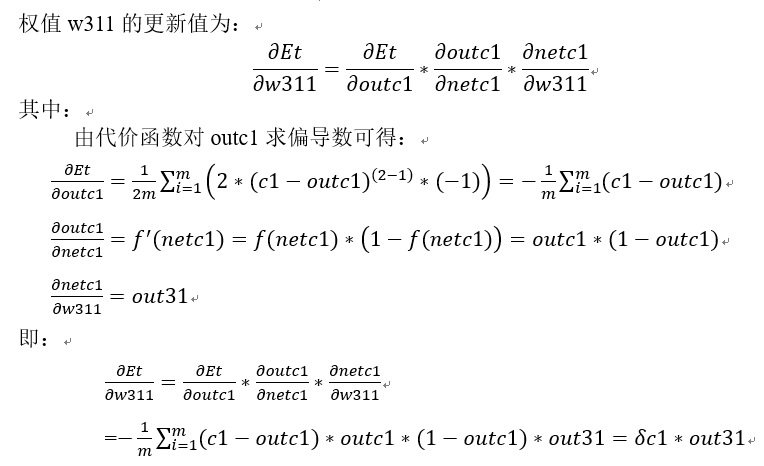(f8)
由上式可见，权值w311是神经网络第3层中第1个神经元至下一层(输出层)的第1个神经元之间的权值；其权值的更新量为第3层中第1个神经元的输出值out31与下一层(输出层)的第1个神经元的误差值之间的乘积。
输出层的第1个神经元的误差值的等于外部信号传至本神经元的误差值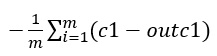(f9)与本神经元输出值对本神经元输入值的导数outc1*(1-outc1)两者的乘积。外部信号传至本神经元的误差值即为代价函数对神经元输出变量的导数。
同理，权值w312的更新量为：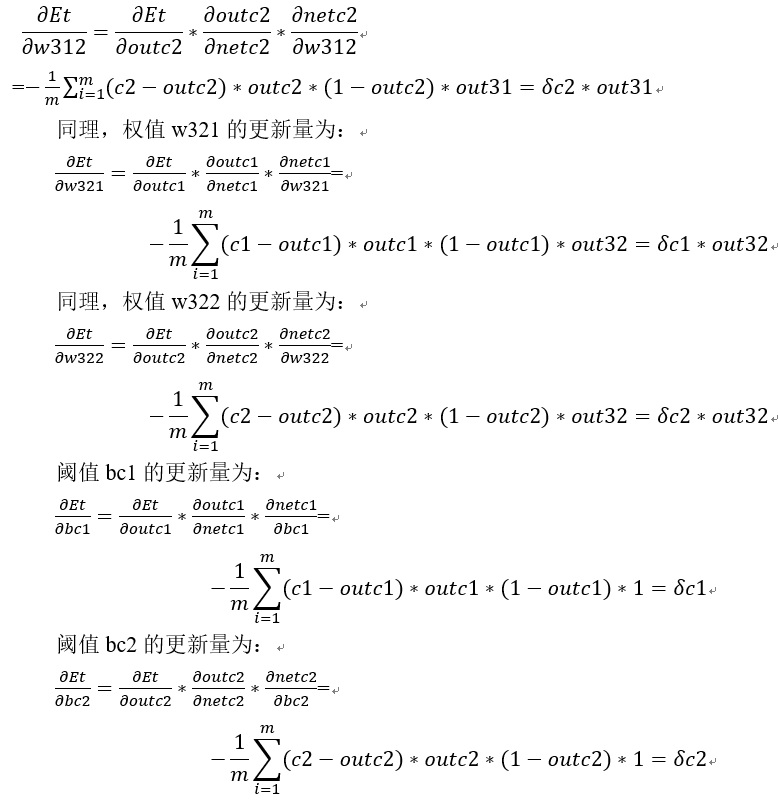(f10)
(3).  第3层权值和阈值更新量反向计算：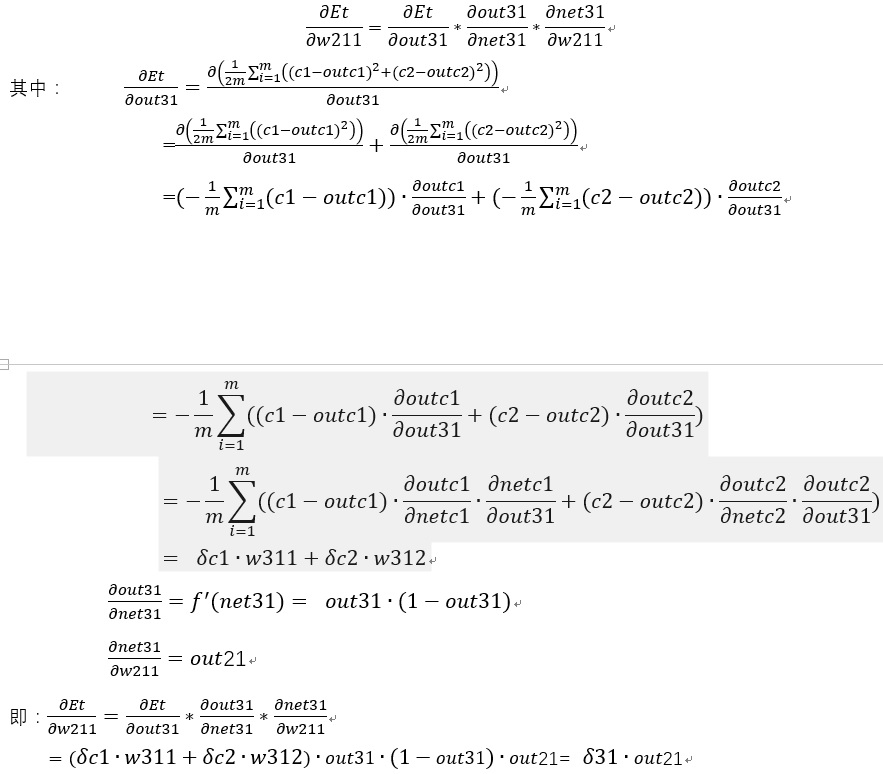(f11)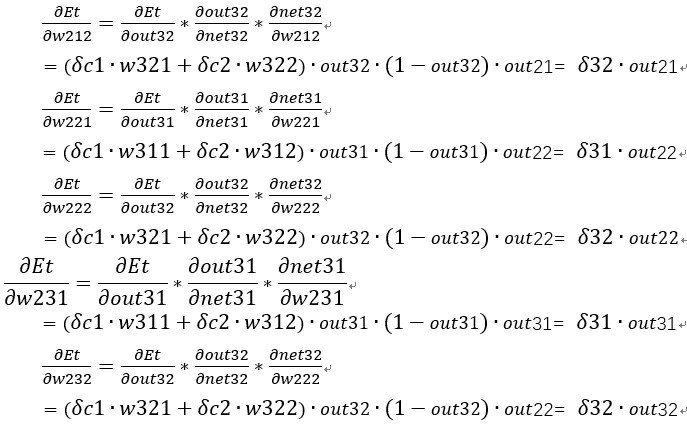(f12)
同理可得阈值的更新量：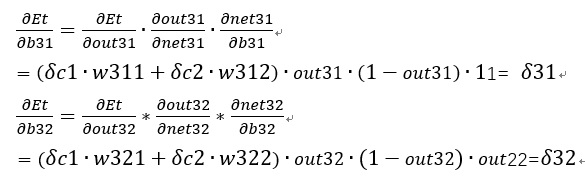(f13)
(4).  第2层权值和阈值更新量反向计算：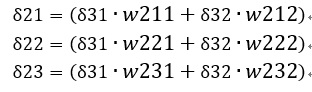(f14)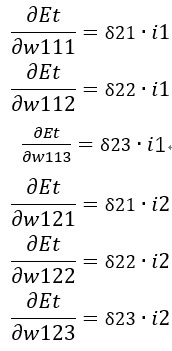(f15)
5.     总结
神经网络某层神经元nxi的误差值包含两大项：
（1）   神经元nxi激活函数的导数：f’(nxi)；
（2）   后一层所有神经元的误差值传递生成本层的误差值之和；上层某个神经元传输至nxi神经元的误差等于上层某个神经元的误差与这两个神经元连接权值的乘积。此处所述的后一层所有神经元包含s个神经元: n(x+1)1,n(x+1)2, n(x+1)3, n(x+1)4,…, n(x+1)5。
某个权值wtki的更新量为wtki终止神经元n(t+1)i的误差值与wtki输入信号值(即outtk)的乘积。T代表w所处的神经元的层数；k代表起始层中神经元的编号；i代表终止层中神经元的编号。
权值的初始化方法决定了梯度下降的过程是否会陷入局部极小的状态。

展开全文神经网络 算法
• 该笔记主要是反向传播公式的推导，理解反向传播的话建议看其他博客中更加具体的例子或者吴恩达老师反向传播介绍的视频（有具体数字的例子），主要有4个公式的推导： (BP1)δjL=∂C∂zjL=∂C∂ajL⋅σ′(zjL)\...
参考：《神经网络与深度学习》
https://legacy.gitbook.com/book/xhhjin/neural-networks-and-deep-learning-zh
该笔记主要是反向传播公式的推导，理解反向传播的话建议看其他博客中更加具体的例子或者吴恩达老师反向传播介绍的视频（有具体数字的例子），主要有4个公式的推导：
$\delta_j^L=\frac{\partial C}{\partial z^L_j}=\frac{\partial C}{\partial a^L_j} \cdot \sigma'(z^L_j) \tag{BP1}$
$\delta ^ {l} = {W^{l+1}} ^\mathsf{T} \cdot \delta^{l+1} \odot \sigma'(z^l) \tag{BP2}$
$\frac{\partial C}{\partial w^{l}_{jk}} = \frac{\partial C}{\partial z^{l}_{j}} \cdot \frac{\partial z^{l}_{j}}{ \partial w^{l}_{jk}} = \delta_j^l \cdot a_k^{l-1} \tag{BP3}$
$\frac{\partial C}{\partial b^{l}_{j}} = \frac{\partial C}{\partial z^{l}_{j}} \cdot \frac{\partial z^{l}_{j}}{ \partial b^{l}_{j}} = \delta_j^l \tag{BP4}$

公式BP1的推导：
先推导神经网络最后一层L的公式，假设一个二分类的神经网络在最后一层如下图所示：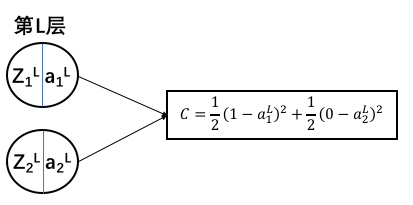C表示损失（或者叫loss）
从公式中可以看出：$\frac{\partial C}{\partial a^L_j}$可直接求出来，接下来是推导$\frac{\partial C}{\partial z^L_j}$, 定义$\delta_j^L=\frac{\partial C}{\partial z^L_j}$

$\delta_j^L=\frac{\partial C}{\partial z^L_j}=\frac{\partial C}{\partial a^L_j} \cdot \sigma'(z^L_j) \tag{1}$
写成矩阵的形式：
$\delta^L = \frac{\partial C}{\partial a^L} \odot \sigma'(z^L) = \Delta_aC \odot \sigma'(z^L) \tag{2}$
公式中$\odot$表示Hadamard积。

公式BP2的推导
L层已经能计算了，接下来是推导前一层的情况，为了表示方便，直接考虑从l+1层到l层的情况，神经网络以及变量命名示意图如下图所示：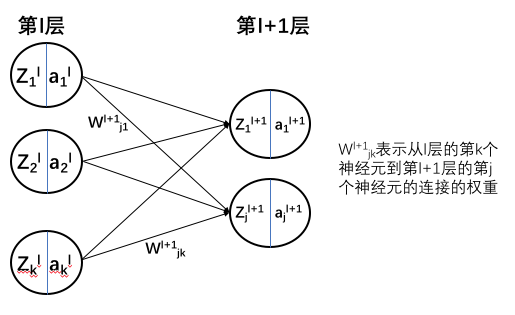（这里$w_{jk}^{l+1}$表示从k神经元到j神经元，理解起来有些拗口，主要是为了方便正向传播中W矩阵的表示，也可以按照自己喜好来进行变量命名，反向传播公式推导麻烦很大程度上是因为变量命令麻烦。）
先得弄清楚前向传播中的一个公式：
$z_j^{l+1}= \sum_{k=1}^k w_{jk}^{l+1} \cdot a_k^l+b_j^{l+1} \tag{3}$

写成矩阵形式为：
$Z^{l+1}= W^{l+1} \cdot A^l+B^{l+1} \tag{4}$
在公式（3）中，现在$\frac{\partial C}{\partial z^{l+1}_j}$已知，需要求$\frac{\partial C}{\partial a^{l}_k}$,自然想到链式求导法则：
$\frac{\partial C}{\partial a^{l}_k}= \sum_{j=1}^j \frac{\partial C}{\partial z^{l+1}_j} \cdot \frac{\partial z^{l+1}_j}{\partial a^{l}_k} \tag{5}$
也可以从物理意义去理解这个公式，$\frac{\partial C}{\partial a^{l}_k}$表示$a^{l}_k$的变化对C的影响大小，$a^{l}_k$可以从$z^{l+1}_j(j=1...J)$来影响C的大小，所以公式（5）中需要有累加。
公式（5）继续化简，这里需要参考公式（3）：
$\frac{\partial C}{\partial a^{l}_k} = \sum_{j=1}^j \delta_j^{l+1} \cdot \frac{\partial z^{l+1}_j}{\partial a^{l}_k} = \sum_{j=1}^j \delta_j^{l+1} \cdot w_{jk}^{l+1} \tag{6}$
$\frac{\partial C}{\partial z^{l}_k} = (\sum_{j=1}^j \delta_j^{l+1} \cdot w_{jk}^{l+1}) \cdot \sigma'(z_k^l) \tag{7}$
写成矩阵形式有：
$\begin{bmatrix} \frac{\partial C}{\partial z^{l}_1} \\ \frac{\partial C}{\partial z^{l}_2} \\ \frac{\partial C}{\partial z^{l}_k} \end{bmatrix} = \begin{bmatrix} w^{l+1}_{11} & w^{l+1}_{21} & w^{l+1}_{j1} \\ w^{l+1}_{12} & w^{l+1}_{22} & w^{l+1}_{j2} \\ w^{l+1}_{1k} & w^{l+1}_{2k} & w^{l+1}_{jk} \\ \end{bmatrix} \cdot \begin{bmatrix} \delta_1^{l+1} \\ \delta_2^{l+1} \\ \delta_j^{l+1} \end{bmatrix} \odot \begin{bmatrix} \sigma'(z_1^l) \\ \sigma'(z_2^l) \\ \sigma'(z_k^l) \end{bmatrix} \tag{8}$
$\begin{bmatrix} \frac{\partial C}{\partial z^{l}_1} \\ \frac{\partial C}{\partial z^{l}_2} \\ \frac{\partial C}{\partial z^{l}_k} \end{bmatrix} = \begin{bmatrix} w^{l+1}_{11} & w^{l+1}_{12} & w^{l+1}_{1k} \\ w^{l+1}_{21} & w^{l+1}_{22} & w^{l+1}_{2k} \\ w^{l+1}_{j1} & w^{l+1}_{j2} & w^{l+1}_{jk} \\ \end{bmatrix} ^\mathsf{T} \cdot \begin{bmatrix} \delta_1^{l+1} \\ \delta_2^{l+1} \\ \delta_j^{l+1} \end{bmatrix} \odot \begin{bmatrix} \sigma'(z_1^l) \\ \sigma'(z_2^l) \\ \sigma'(z_k^l) \end{bmatrix} \tag{9}$
$\delta ^ {l} = {W^{l+1}} ^\mathsf{T} \cdot \delta^{l+1} \odot \sigma'(z^l) \tag{10}$
3.公式BP3的推导：
然后推导$\frac{\partial C}{\partial w^{l}_{jk}}$ 和 $\frac{\partial C}{\partial b^{l}}$, 这也是神经网络中实际参数更新需要计算的参数，先推导$\frac{\partial C}{\partial w^{l}_{jk}}$。
根据公式（3）可知：
$\frac{\partial C}{\partial w^{l}_{jk}} = \frac{\partial C}{\partial z^{l}_{j}} \cdot \frac{\partial z^{l}_{j}}{ \partial w^{l}_{jk}} = \delta_j^l \cdot a_k^{l-1} \tag{11}$
4.公式BP4的推导：
然后推导$\frac{\partial C}{\partial b^{l}}$
$\frac{\partial C}{\partial b^{l}_{j}} = \frac{\partial C}{\partial z^{l}_{j}} \cdot \frac{\partial z^{l}_{j}}{ \partial b^{l}_{j}} = \delta_j^l \tag{12}$


展开全文深度学习 人工智能 机器学习
• https://zhuanlan.zhihu.com/p/23270674 https://www.zybuluo.com/Feiteng/note/20154 转载于:https://www.cnblogs.com/ymjyqsx/p/6709036.html
• 深度学习多分类问题中，最后一层通常会用Softmax输出每个类别的概率，然后计算交叉熵...上述两种情况加起来便可以得到最终的反向传播公式： 参考： https://blog.csdn.net/Charel_CHEN/article/details/81266575 ...深度学习 人工智能 机器学习
• ResNet残差网络结构在深层网络中训练效率会提高，为什么呢，我们下面用公式...1、l层全连接层的反向传播的W的梯度公式   2、l层全连接层的反向传播的b的梯度公式   3、层与层之间的梯度传播依靠到的过渡   ...ResNet 残差网络 梯度
• 前言：最近在深度学习中，了解到多分类的softmax算法，且利用该算法在进行反向传播时除了损失函数与...这里主要对softmax算法的反向传播求解dZ进行了公式推导。其他部分与sigmoid算法求解一致。 符号说明 ...机器学习 softmax
• 记住这三项计算公式，后面的工作都以这几个公式为指导依次展开 误差项的递归计算： tips： 在最后一个时间帧（T）上的计算 ，输出层的计算误差项： 隐含层： 这里的求导使用了链式求导法则，并且上一步求过的到vt的...RNN
• 反向传播公式，主要是链式法则，找了几篇博客，详细推导过程和示例计算都有。 https://blog.csdn.net/qrlhl/article/details/50885527 https://www.jianshu.com/p/408ab8177a53 ...
• 深度神经网络反向传播公式推导 反向传播推到 上边连接推到的很详细。
• 在神经网络反向传播公式这一节，吴恩达只给出了公式结果，没有给出公式推导过程。 关于公式推导过程，我找到一篇还不错的博客，地址如下： https://blog.csdn.net/xuan_liu123/article/details/83660316 但是这篇...机器学习 神经网络
• 池化层反向传播公式推导 池化层在深度学习网络架构中的作用包括: * 减少计算量 减少特征图尺寸,减少后面的层的计算量 提高多尺度信息 如果存在多个池化层,就相当于网络中构造了一个多尺度特征金字塔,多尺度...池化层
• 原文链接：Batch Normalization反向传播公式的推导 代码部分是CS231n的作业。看完推导公式配合作业来加深理解。上面原文已经推导得很清楚了。看原文即可。 再附一篇好的博文:Batch Normalization学习笔记及其实现 ...
• 梯度下降及反向传递公式推导 之前发布在作业部落了，可以通过这个链接访问，其中的数学公式转过来比较麻烦，先看作业部落的链接吧：https://www.zybuluo.com/JackMeGo/note/1052246 ...梯度下降
•梯度下降 神经网络
• 复合函数求导法则： 对神经网络的代价函数进行求导的时候，使用反向传播算法计算，会用到上述法则 前向传播公式： 假定: 不定义偏置b 激活函数为a 则有： = a ( * ) 总结： 本层输出 = 激活函数 （ 本层参数 * 本层...神经网络
• http://courses.cs.tau.ac.il/Caffe_workshop/Bootcamp/pdf_lectures/Lecture%203%20CNN%20-%20backpropagation.pdf http://courses.cs.tau.ac.il/Caffe_workshop/Bootcamp/pdf_lectures/ ...
• 单隐含层前馈神经网络前向传播及反向传播公式推导如下
• 最近学习了吴恩达老师的deeplearning.ai中的神经网络与深度学习课程，将其中2.13节介绍的Logistic回归原理及反向传播公式进行推导，具体如下
• ## 神经网络的反向传播公式的推导

千次阅读 多人点赞 2018-11-28 01:31:55
神经网络的反向传播公式的推导 前言： 早该开始入坑CNN,RNN的博主总觉得要先能用python加numpy手撸一个神经网络，才能更好理解其他神经网络的原理（强迫症）。于是…这一拖就是快两月（懒），最近填坑的时候才发现...
• sigmoid之所以被relu替代,就是relu梯度为1(输入大于0时),不会压缩梯度,反向传播时更加有利 假设已知网络输出 J J J 对 z k j z j k z^k_j 的导数 δ k j = d J d z k j δ j k = d J d z j k \delta _j^k =\frac...
• 机器学习算法工程师的面试中常会问到一个很基础的问题，那就是反向传播公式的推导，今天看了下吴恩达老师的公开课《神经网络和深度学习》，将一些推导过程记录下来。 逻辑回归反向传播的推导 逻辑回归是最简单的...深度学习 神经网络
• 感知机的反向传播公式推导 输入为x=[x1,x2,⋯ ,xn]T\textbf{x} = \left[ x_1, x_2, \cdots, x_n \right]^Tx=[x1​,x2​,⋯,xn​]T，权重为w=[w1,w2,⋯ ,wn]T\textbf{w} = \left[ w_1, w_2, \cdots, w_n \right]^...1024程序员节 深度学习
• 本着好读书不求甚解的精神，本文只讨论对BP四公式使用的理解，推导请自行百度，给两篇参考博客1，博客2，两者结合效果更佳。 二、大结局 只有能用自己的话解释公式，才算理解了它。 ----鲁迅 BP四公式 缭乱的......